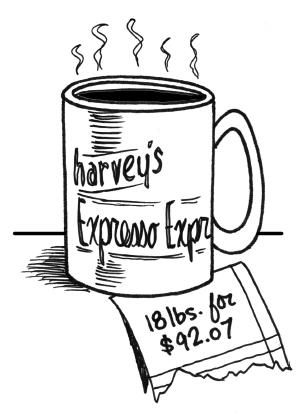### Home > INT3 > Chapter 2 > Lesson 2.2.1 > Problem2-35

2-35.

Harvey’s Espresso Express, a drive-through coffee stop, is famous for its delicious house coffee, a blend of Colombian and Mocha Java beans. Their archrival, Jojo’s Java, sent a spy to steal their ratio for blending beans. The spy returned with a torn part of an old receipt that showed only the total number of pounds and the total cost, $18$ pounds for $\92.07$. At first Jojo was angry, but then he realized that he knew the price per pound of each kind of coffee ($\4.89$ for Colombian and $\5.43$ for Mocha Java). Show how he could use equations to figure out how many pounds of each type of beans Harvey’s used to make its house coffee.

First, define variables you can use to write equations.
Let $c$ = the number of pounds of Colombian coffee beans
Let $m$ = the number of pounds of Mocha Java coffee beans

Next, set up an equation to represent the information about cost.

$4.89c+5.43m=92.07$

Now set up a second equation to show the total number of pounds.

Finally, solve your system of equations.